# 一次失败的抛物线正等测投影变换，以及什么情况下才能放弃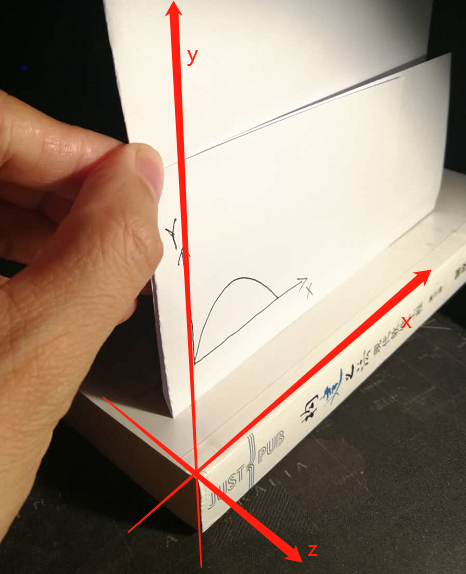# 1.问题

## 1.1 跳一跳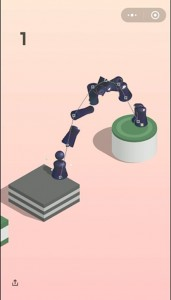# 2.涉及的概念

## 2.1 投影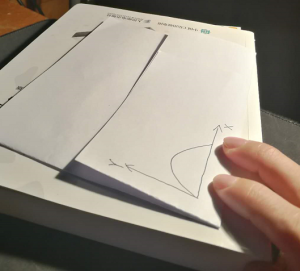但是人类可以借由脑补想像出这张纸上画了一条抛物线，不是近大远小的，而是如下图这样。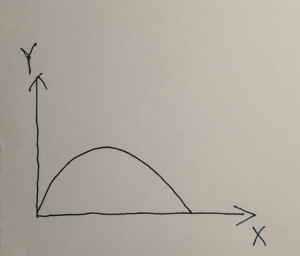## 2.2 正等测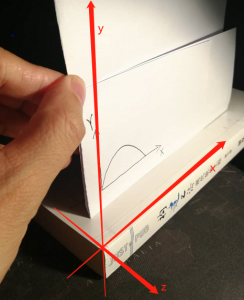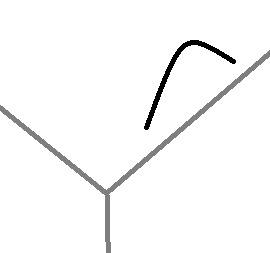根据正等测的定义[https://en.wikipedia.org/wiki/Isometric_projection]，对抛物线的每个点做如下操作: (1)先绕y轴旋转 45度，(2)然后绕x轴35.264度，(3)最后向xy平面投影。35.264度这个有零有整的数字，是因为arcsin(tan 30°)大约是这个角度，目的是原来的坐标轴完成两次旋转后，刚好与原本的正方体不在同一表面上的两个顶点(下图中的红线连接的两个顶点)重合。也就是说，正等测是从这两个顶点的连线的角度，即这两个顶点看起来刚好是重合的。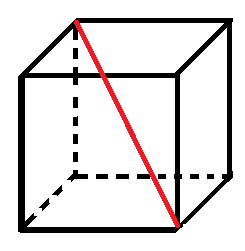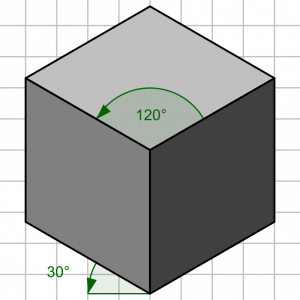### 2.3 变换矩阵

《计算机图形学》教材上提到，二维/三维图形的平移、旋转、扭转、错切等变换，都可以通过对空间中的点的齐次坐标做矩阵乘法完成。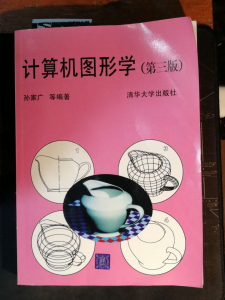### 2.3.1 点的变换

(x y z 1)，以下矩阵乘法能完成关于 Z 轴进行对称变换。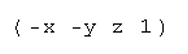使用矩阵的好处是所有变换运算都是矩阵乘法，不必每个单独考虑，需要哪个变换就用对应的矩阵。

### 2.3.2 隐函数形式曲线的变换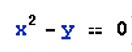这一方程可以表示为矩阵乘法的形式，下面3个矩阵相乘的结果就是上式的等号左侧，x^2-y。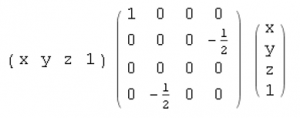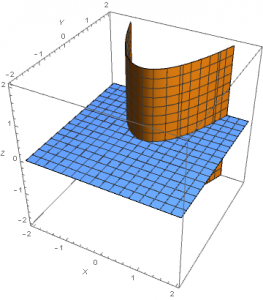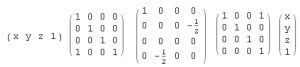(1) 三维齐次坐标 矩阵和方程间的转换

```In:= A =
{{a, b/2, d/2, h/2}, {b/2, c, e/2, j/2}, {d/2, e/2, g,  k/2}, {h/2, j/2, k/2, f}}
Out= {{a, b/2, d/2, h/2}, {b/2, c, e/2, j/2}, {d/2, e/2, g, k/2}, {h/2, j/2, k/2, f}}
In:= Expand[L.A.R]
Out= {{f + h x + a x^2 + j y + b x y + c y^2 + k z + d x z + e y z + g z^2}}```

(2)矩阵及矩阵转置连乘的几何意义

`L.RZ.A.Transpose[RZ].Transpose[L]`

L是{x,y,z,1}，几何意义是任意一个点。

L.RZ 得到 {x’,y’,z’,1}，是这个点的变换。

Transpose[RZ].Transpose[L]，Transpose[L]左乘RZ的转置，几何上等价于于Transpose[L]右乘RZ，得到{x’,y’,z’,1}的转置。

`L.RZ.A.Transpose[RZ].R`

` {x',y',z',1}.A.Transpose[{x',y',z',1}]`

{x’,y’,z’,1}.A.Transpose[{x’,y’,z’,1}]与L.A.R的形式相同，

L.A.R，根据矩阵乘法得到 f(x,y,z)。

```In:= A =
{{a, b/2, d/2, h/2}, {b/2, c, e/2, j/2}, {d/2, e/2, g, k/2}, {h/2, j/2, k/2, f}}
Out= {{a, b/2, d/2, h/2}, {b/2, c, e/2, j/2}, {d/2, e/2, g, k/2}, {h/2, j/2, k/2, f}}
In:= Expand[L.A.R]
Out= {{f + h x + a x^2 + j y + b x y + c y^2 + k z + d x z + e y z + g z^2}}```

### 2.3.3 参数方程形式曲线的变换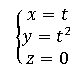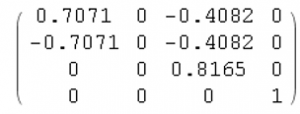`tf = {t, t^2, 0, 1}`

`T = {{0.7071, 0, -0.4082, 0}, {-0.7071, 0, -0.4082, 0}, {0, 0, 0.8165,0}, {0, 0, 0, 1}}`

`tf.T`

`{0.7071 t - 0.7071 t^2, 0, 0. - 0.4082 t - 0.4082 t^2, 1}`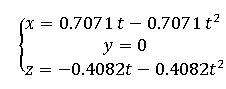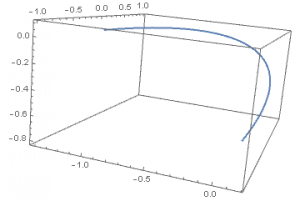注:参数方程的方法是由典同学提供的。在请教他以前，我把“参数图表”误读为参数方程，已尝试了9个小时以上。典同学花了20分钟不到，给出了上述方案，这20分钟还包括学习正等测是什么，以及复杂mathematica语法的时间。没有他的指导，我还在隐函数的沼泽挣扎。事实上，我又花了10小时以上，想找到隐函数的可行之路，直到认定这条技术路线不可能。所以，才有了这篇博客，目的是给出失败的过程，以及说明在什么情况下才能认定可以放弃。

# 3. 技术路线

(1)三维空间中的抛物线ab0是抛物面a0与平面b0的交线。特别的，在跳一跳中，是z值任意的抛物面a1与z==0平面即XOY平面b1的交线。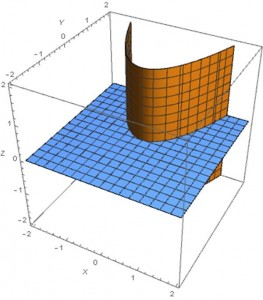(2)对抛物面a1做正等测(前两步，绕轴旋转不投影)变换得到a2; 对XOY平面b1做正等测(前两步，绕轴旋转不投影)变换得到b2。

(3)把a2与b2的交线投影到XOY平面，所得曲线ab3为a0与b0的交线的正等测投影。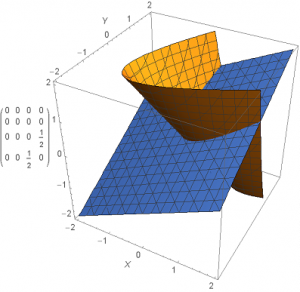## 4.1 抛物面

```L={{x,y,z,1}}
R={{x},{y},{z},{1}}
A={{a,b/2,0,d/2},{b/2,c,0,e/2},{0,0,0,0},{d/2,e/2,0,f}}
a=1;b=0;c=0;d=0;e=-1;f=0
ContourPlot3D[{L.A.R==0},{x,-2,2},{y,-2,2},{z,-2,2},AxesLabel->{X,Y,Z}]```

## 4.2 XOY平面

```Z={{0,0,0,0},{0,0,0,0},{0,0,0,1/2},{0,0,1/2,0}}
ContourPlot3D[{L.Z.R==0},{x,-2,2},{y,-2,2},{z,-2,2},AxesLabel->{X,Y,Z}]```

##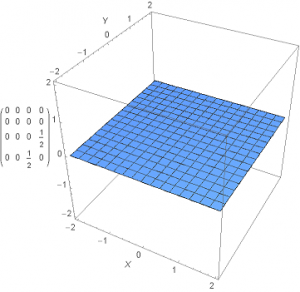4.3 抛物面与平面相交的几何直观

`ContourPlot3D[{L.Z.R==0,L.A.R==0},{x,-2,2},{y,-2,2},{z,-2,2},AxesLabel->{X,Y,Z}]`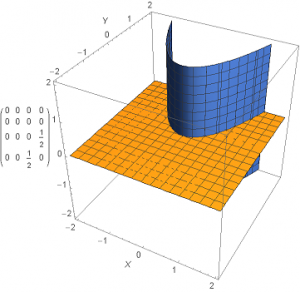## 4.4 验证矩阵乘法实现平移变换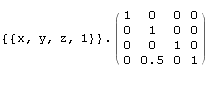在Mathematica中，输入如下代码，等价于上面的矩阵相乘。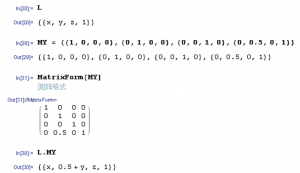所得结果{{x, 0.5 + y, z, 1}}表明，纵坐标数值增加0.5，即偏移0.5。实验结果旁证用矩阵乘法与平移是等价的。

## 4.5 隐函数的变换

```In:= MX = {{1, 0, 0, 0}, {0, 1, 0, 0}, {0, 0, 1, 0}, {2, 0, 0, 1}}
Out= {{1, 0, 0, 0}, {0, 1, 0, 0}, {0, 0, 1, 0}, {2, 0, 0, 1}}
In:= L.A.Transpose[L]
Out= {{x^2 - y}}
In:= Expand[L.MX.A.Transpose[MX].Transpose[L]]
Out= {{4 + 4 x + x^2 - y}}```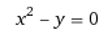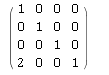变换后即(用[https://www.wolframalpha.com/]绘制)

## 4.6 隐函数的变换的复合

L.A.R

L.T.A.Traspose[T].R

L.M1.RX.M2.A.Traspose[M2]. Traspose[RX].Traspose[M1].R

(我不知道什么是“基本”运算，求助。)

## 4.7 正等测变换矩阵分解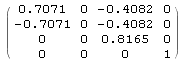代码形式MatrixForm[{{0.7071, 0, -0.4082, 0}, {-0.7071, 0, -0.4082, 0}, {0, 0, 0.8165, 0}, {0, 0, 0, 1}}]。

RZ = {{1/Sqrt, 1/Sqrt, 0, 0}, {-(1/Sqrt), 1/Sqrt, 0, 0}, {0, 0, 1, 0}, {0, 0, 0, 1}}

RX  = {{1, 0, 0, 0}, {0, Sqrt[2/3], -(1/Sqrt), 0}, {0, 1/Sqrt, Sqrt[2/3], 0}, {0, 0, 0, 1}}

PXY = {{1, 0, 0, 0}, {0, 0, 0, 0}, {0, 0, 1, 0}, {0, 0, 0, 1}}

N[MatrixForm[RZ.RX.PXY]]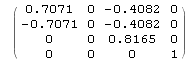因此，两矩阵相同，因此正等测变换可以分解为三个运算的复合。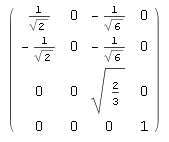## 4.8 正等测变换矩阵实例

(1)原始图形为抛物面与XOY平面。

ContourPlot3D[{L.A.Transpose[L]==0, L.Z.Transpose[L]==0},{x,-2,2},{y,-2,2},{z,-2,2},AxesLabel->{X,Y,Z}]

ContourPlot3D[{L.RZ.A.Transpose[RZ].Transpose[L]==0, L.RZ.Z.Transpose[RZ].Transpose[L]==0},{x,-2,2},{y,-2,2},{z,-2,2},AxesLabel->{X,Y,Z}]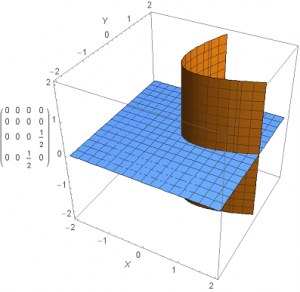我们注意到XOY平面相对Z旋转时，变换结果与原图相同。XOY平面与抛物面交线所得抛物线的轴由原图中的平行于Y轴旋转为穿过图中(2,2,0)，转过45度。

(2)绕X轴(图中为横轴) 旋转arcsin(tan 30°)约合264度。

ContourPlot3D[{L.RZ.RX.A.Transpose[RX].Transpose[RZ].Transpose[L]==0, L.RZ.RX.Z.Transpose[RX].Transpose[RZ].Transpose[L]==0},{x,-2,2},{y,-2,2},{z,-2,2},AxesLabel->{X,Y,Z}]我们注意到原图中蓝色的XOY平面穿过右上角顶点(2,2,2)和(-2,-2,-2)。从对角线(2,2,2)到(-2,-2,-2)看去，蓝色平面是一条直线。下图为上图手动旋转变换视角，可以看到蓝色平面看起来是右上到左下的直线。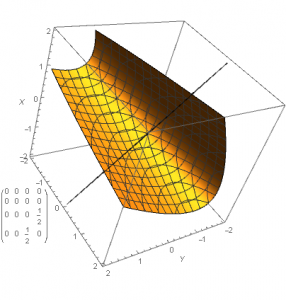下图中的绿线是手动标注的，从这个视角看，得到抛物面和蓝色原XOY平面的的交线在XOZ平面的投影。这一投影即原图中抛物线 y=x^2 在正等测结果。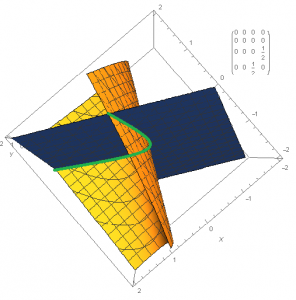## 4.9 交线方程组的矩阵表示

Z平面做正等测前两步(两次旋转，不含投影到XOY)得到矩阵可以表达为方程(我们称为a)。

(1)方程两侧分别相减、相乘

(2)解出每个方程的根，然后令这些根之间相等

(3)换个维度

(4)引入参数方程

(5)各种交线投影

(6)只画线，不求解方程组

# 5. 障碍小结

RH同学提到过某个项目非常快的失败了，挺遗憾的感觉。在我看来，项目能快点失败是件好事，避免消耗更多的资源，如果最终仍然会失败的话。工程是以逐利为目的，这是工程上纯功利的看法。如果会失败，请尽快。除此以外，失败的项目才是主流，大多数项目都或多或少失败了，而完成成功的非常少。这些失败带给我们的教益，远比成功要大。即使你按成功的项目的方法再来一次，也不见得成功。但是你按失败的方法再来一次，一定失败。所以对于失败的认识非常可靠，可靠地指导我们未来的实践。

GYB同学

DQT同学

LDT同学

# 7. 参考资料

孙家广: 计算机图形学(第三版)，清华大学出版社

GYB同学分别给出的数值解和解析解求法

[https://www.cnblogs.com/gaoyb348/p/9042867.html]

当你说不行时，你应该说些什么：以Android播放midi为例

郭绍仲.轴测投影变换矩阵的研究[J].工程图学学报,1989,(2):17-24.

[5 ]计算机图形学 学习笔记（八）：三维图形变换：三维几何变换，投影变换（平行/ 透视 投影）
[https://blog.csdn.net/jurbo/article/details/75041230]

——————————————————